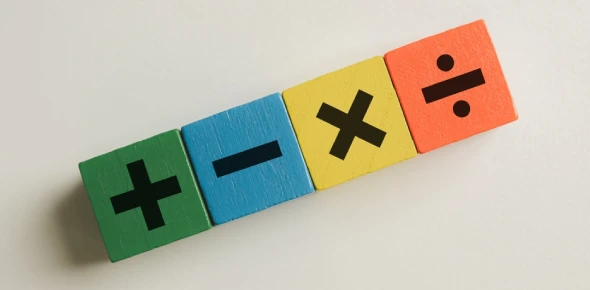# Galderma Trade Maths & Profitability

Approved & Edited by ProProfs Editorial Team
The editorial team at ProProfs Quizzes consists of a select group of subject experts, trivia writers, and quiz masters who have authored over 10,000 quizzes taken by more than 100 million users. This team includes our in-house seasoned quiz moderators and subject matter experts. Our editorial experts, spread across the world, are rigorously trained using our comprehensive guidelines to ensure that you receive the highest quality quizzes.
| By Gezzar
G
Gezzar
Community Contributor
Quizzes Created: 2 | Total Attempts: 1,377
Questions: 5 | Attempts: 77SettingsFollow up questions to the workshop on 1st May, 2012 facilitated by Gerald Richards of Market People
Yes! As promised and what you have been waiting for with bated breath. The follow up questions for the next 16 weeks!
I receive a report on your answers which allows me to gauge the effectiveness of the training.
Please take the time to "have a go" so you can develop your skills and confidence through practice. Am happy to receive any questions or comments as feedback is most important.
Have fun.
Kind Regards
Gerald Richards
Market People

• 1.

### How many different sizes of packaging are there for Cetaphil Gentle Cleanser?

• A.

1

• B.

2

• C.

3

• D.

4

• E.

5

• F.

6

D. 4
Explanation
125ml, 250ml, 500ml and 1Litre

Rate this question:

• 2.

### Pharmacy buys Cetaphil Gentle Cleanser 125ml at wholesale of \$4.48 (ex GST) and sells for \$7.58 (inc GST). Calculate \$Profit.

\$2.41
2.41
Explanation
The profit can be calculated by subtracting the cost price from the selling price. In this case, the cost price of the Cetaphil Gentle Cleanser is \$4.48 (ex GST) and the selling price is \$7.58 (inc GST). Therefore, the profit is \$7.58 - \$4.48 = \$3.10. However, since the answer options only include \$2.41, the correct answer is \$2.41.

Rate this question:

• 3.

### Pharmacy buys Cetaphil Gentle Cleanser 125ml at wholesale of \$4.48 (ex GST) and sells for \$6.89 (ex GST). Calculate %Margin and %Mark up

• A.

%Mark up = 35.79%

• B.

%Margin = 38.89%

• C.

%Mark up = 53.79%

• D.

%Margin = 43.98%

• E.

%Mark up = 53.97%

• F.

%Margin = 34.98%

C. %Mark up = 53.79%
F. %Margin = 34.98%
Explanation
The %Mark up is calculated by subtracting the cost price from the selling price, dividing the result by the cost price, and then multiplying by 100. In this case, the cost price is \$4.48 and the selling price is \$6.89. The difference between the selling price and the cost price is \$2.41. Dividing \$2.41 by \$4.48 and multiplying by 100 gives a %Mark up of 53.79%.

The %Margin is calculated by subtracting the cost price from the selling price, dividing the result by the selling price, and then multiplying by 100. In this case, the difference between the selling price and the cost price is \$2.41. Dividing \$2.41 by \$6.89 and multiplying by 100 gives a %Margin of 34.98%.

Rate this question:

Related TopicsBack to top